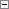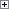Use this simple data type to store a single, 8-bit character as a value in the range 0 to 255. You can easily convert this data type from a number to a character and vice versa. This means you can use mathematical operators on Byte variables.Example

The following example assumes that you have a Byte variable named B and a Text variable named S.

You can assign a constant string of the length 1 to a Byte variable, as shown in the first line of the following code example.

You can assign a single character in a Text or Code variable to a Byte variable, as shown in the second line of the following code example.

You can assign a numeric value to a Byte variable, as shown in the third line of the following code example. This causes the Byte variable to contain the character from the ASCII character set that corresponds to the numeric ASCII code.Copy Code
B := 'A';
B := S;
B := 65;

You cannot assign a character to a position greater than the position of the null terminator. For example, if the value of the text variable MyText is 'abc', then the null terminator is at position 4 and the following assignment causes a run-time error to occur.Copy Code
MyText := 'e';